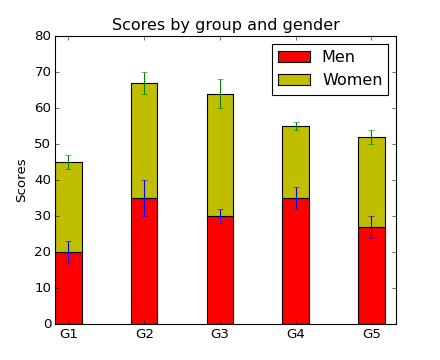### We're updating the default styles for Matplotlib 2.0

Learn what to expect in the new updates#### Previous topic

pylab_examples example code: axis_equal_demo.py

#### Next topic

pylab_examples example code: barb_demo.py

# pylab_examples example code: bar_stacked.py¶```#!/usr/bin/env python
# a stacked bar plot with errorbars
import numpy as np
import matplotlib.pyplot as plt

N = 5
menMeans   = (20, 35, 30, 35, 27)
womenMeans = (25, 32, 34, 20, 25)
menStd     = (2, 3, 4, 1, 2)
womenStd   = (3, 5, 2, 3, 3)
ind = np.arange(N)    # the x locations for the groups
width = 0.35       # the width of the bars: can also be len(x) sequence

p1 = plt.bar(ind, menMeans,   width, color='r', yerr=womenStd)
p2 = plt.bar(ind, womenMeans, width, color='y',
bottom=menMeans, yerr=menStd)

plt.ylabel('Scores')
plt.title('Scores by group and gender')
plt.xticks(ind+width/2., ('G1', 'G2', 'G3', 'G4', 'G5') )
plt.yticks(np.arange(0,81,10))
plt.legend( (p1, p2), ('Men', 'Women') )

plt.show()
```

Keywords: python, matplotlib, pylab, example, codex (see Search examples)